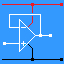RANDOM PAGE

SITE SEARCH

LOG
IN

HELP

# Op Amp Gain

This is the AQA version closing after June 2019. Visit the the version for Eduqas instead.

## Amplifiers increase the magnitude of the input signal.

Gain is a number (with no units) used to describe how much bigger a signal becomes when it is amplified.## Gain Calculations

• Voltage Gain = Vout / Vin

• Current Gain = Currentout / Currentin

• Power Gain = Powerout / Powerin

## Amplifiers have one or more of the following properties

• The output voltage may be bigger than the input voltage
• The output current may be bigger than the input current
• The output power may be bigger than the input power
• High input impedance (AC equivalent of resistance)
• Low output impedance (AC equivalent of resistance)

## Voltage Bandwidth

This is the range of frequencies where the amplifier output VOLTAGE is at least 70% of the maximum amplifier output.

The graph shows the bandwidth going from 1Hz up to 1 MHz.## Power Bandwidth

This is the range of frequencies where the amplifier output POWER is at least 50% of the maximum amplifier output.

The graph shows the bandwidth going from 1Hz up to 1 MHz.## Examples

Microphone Pre-amplifier

This has a high input impedance, a low output impedance and a useful voltage gain. The gain needed depends on the type of microphone and on whether the power amplifier sub-system provides any additional voltage gain.

Voltage Follower used as a Voltage Regulator

A Zener diode is used to provide a reference voltage. The voltage regulator provides any required output current up to its rated maximum without the output voltage significantly changing. The voltage gain is 1.

Power Amplifier (audio or radio frequency)

This sub-system can have a voltage gain as low as 1 but the output current is generally much larger than the input current.

Push Pull Amplifier

One MOSFET or transistor amplifies the positive half of the input signal. A second MOSFET or transistor amplifies the negative half of the input signal. The outputs from both devices are combined. This is also called Class B amplification.

• This has a 50 or 75 Ohm or sometimes a high input impedance.
• It usually has a similar output impedance.
• It provides voltage and/or power gain. It is used to
• boost weak radio signals before they are processed by later sub-systems in a receiver
• increase the output power of a transmitter (possibly up to megawatt levels(interesting electronics here))

reviseOmatic V3     Contacts, ©, Cookies, Data Protection and Disclaimers Hosted at linode.com, London